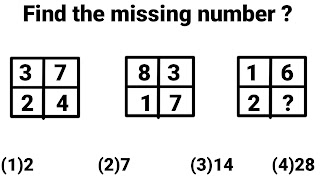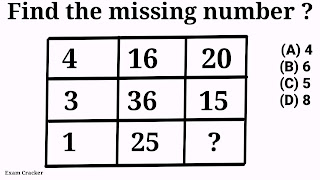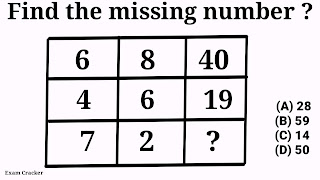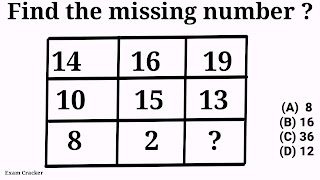## Wednesday, 1 June 2022

### Missing number in box Reasoning, Missing number in box puzzle

Ten most important missing number in box Reasoning, Missing number in box puzzle will be discussed with the help of ten most important examples. Some of these examples are of  3 × 3  order and other are of  4 × 4 orders.

# Reasoning of missing number in box problems

## Problem # 1Exam Cracker
This box problem consist of four rows and four columns . And we have to find the value of question mark after studying the pattern of all the numbers in this box. This question mark is in 4th row of 4th column.
To find the value of question mark. we  shall divide this box into two parts vertically then we can have the formula for these numbers written in this box . Because after careful observation we can see that the product of both the numbers in left half in any particular row is equal to sum of both the numbers in right half in that particular row.
Column wise :-
Formula:-  (1st number × 2nd number ) = (3rd number × 4th number)
15 × 10 = 6 × 25 = 150 (Equal Product in both the half of 1st row)
4  × 16  =  8 × 8 = 64 (Equal Product in both the half of 2nd row)
6  ×  6 = 12 × 3 =  36 (Equal Product in both the half of 3rd row)
Similarly
7 × 9 = 3 ×  ? = 63 (Equal Product in both the half of  4th row)
? = 63
⇒  ? = 21
Option (B)21 is correct option.

## Problem # 2Exam Cracker

This reasoning problem consists of three figures and every figure have four numbers associated to it . Two numbers are on the upper line of each box and two number are at the bottom line of each box.  Look at last figure , it have ? in its 3rd figure . So the solution of this problem is to find the value of question mark using three numbers associated to it .

But the main problem is how to utilised  these three numbers to get the value of question mark?
Now watch carefully the 1st two figures . Since these figures have some big values of numbers in one box .
Now we have to find or search the  formula for these four numbers in 1st two figures to utilised them in any possible way to get number in that box .
The same formula will be applicable to third figure to find out the value of question mark.
Formula:-  Product of all the numbers = 168

1st Box

Product of  all the numbers in 1st box is equal to 168

3 × 7 × 4 × 2 = 168 (1st figure)

2nd Box

Product of  all the numbers in 2nd box is equal to 168

8 × 3 × 7 × 1 = 168 (2nd figure)

3rd Box

Product of  all the numbers in 2nd box is equal to 168

1 × 6 × ? × 2 = 168 ( 3rd figure )

12 × ? = 168

? = 168 ÷ 12
? =  14
Option (3)14 is correct option.

## Problem # 3Exam Cracker

This box problem consist of four rows and three columns . And we have to find the value of question mark after studying the pattern of all the numbers in this box. This question mark is in 4th row of 3rd column.
To find the value of question mark. we  shall divide this box into two parts horizontally then we can have the formula for these numbers written in this box . Because after careful observation we can see that the product of both the numbers in upper half in any particular column is equal to sum of both the numbers in lower half in that particular column.
Formula:-  (1st number + 2nd number )  =  (3rd number + 4th number)
1st column :-
8 + 7 = 2 + 13 = 15 (Equal sum in both the half of 1st column)
2nd column :-
3 + 6 = 4 + 5 =  9  (Equal sum in both the half of 2nd column)
3rd column :-
4 + 1 = 4 + ? = 5  (Equal sum in both the half of 3rd column)
⇒ 4 + ? = 5
⇒ ? = 5 - 4
⇒ ? = 1
Option (B)1 is correct option.

## Problem # 4Exam Cracker

This box problem consist of three rows and three columns. And we have to find the value of question mark in 3rd row and 3rd column after studying the pattern of all the numbers in this box.
Third number of every row is equal to the sum of square of 1st number and square root of 2nd number.
Formula:-  Square of 1st number +  Square root of 2nd number
(4)2    +  √16 = 16 + 4 = 20  (Number in 1st row 3rd column )
(3)2    +  √36 = 9 + 6 = 15  (Number in 2nd row 3rd column )
(1)2    +  √25 = 1 + 5 = 6  (Number in 3rd row 3rd column )
Option (B)6 is correct option.

##This box problem consist of three rows and three columns. And we have to find the value of question mark after studying the pattern of all the numbers in this box.
1st number of every row is equal to the product of square of 3rd number and square root of 2nd number.

Formula:-  Square of 3rd number  ×  Square root of  2nd number

(3)2    ×  √49 = 9 × 7 = 63 (Number in 1st row 1st column )
(4)2    × √25 = 16 × 5 = 80 (Number in 2nd row 1st column )
(?)2    ×  √64 = 200 (Number in 3rd row 1st column )
(?)2    ×  8 = 200
(?)2   = 200 ÷ 8
(?)2   = 25
?   = 5
Option (D)5 is correct option.

## Problem # 6Exam Cracker

This box problem consist of three rows and three columns. And we have to find the value of question mark after studying the pattern of all the numbers in this box.
Since question mark is in the 3rd column of 3rd row, so we can use any two numbers in any two particular rows  to find the value of 3rd  number in that particular row.

Formula:-  Square of 1st number  +  (2nd number ÷ 2 ) = 3rd number
(6)2   +  ( 8 ÷ 2 ) = 36 + 4 =  40 ( Number in 1st row 3rd column )
(4)2   +  (  6 ÷ 2 ) = 16 + 3 =  19 ( Number in 2nd row 3rd column )
(7)2   +  (  2 ÷ 2 ) = 49 + 1 = 50 ( Number in 3rd row 3rd column )
Option (D)50 is correct option.

## Problem # 7Exam Cracker

This box problem consist of three rows and three columns. And we have to find the value of question mark after studying the pattern of all the numbers in this box.
Since question mark is in the 1st column of 3rd row, so we can use any two number in any two particular columns to find the value of 3rd  number in that particular column.

Formula:-  cube of 2nd number  ×  ( cube root of 3rd number ) = 1st number
(4)3  ×  ∛8 = 64 × 2 = 128 ( Number in 1st row 1st column )
(3)3   ×  ∛64 = 27 × 4 = 108 ( Number in 2nd row 1st column )
(2)3   ×  512 = 8 × 8 = 64 ( Number in 3rd row 1st column )
Option (A)64 is correct option.

## Problem # 8Exam Cracker

This box problem also consist of three rows and three columns. And we have to find the value of question mark after analysing the pattern of all the numbers in this box.
Since question mark is in the 3rd column of 3rd row, so we can use any two number in any two particular rows to find the value of 3rd  number in that particular row.
Row wise
Formula:-  2 × (1st number - 2nd number ) 3rd number
( 14  - 10 ) × 2 = 4 × 2 = 8 ( Number in 3rd row 1st column)
( 16  - 15 ) × 2 = 1 × 2 = 2 ( Number in 3rd row 1st column)
( 19  - 13 ) × 2 = 6 × 2 =  ? = 12 ( Number in 3rd row 1st column)
Option (D)12 is correct option.

## Problem # 9Exam Cracker

This box problem consist of three rows and three columns. And we have to find the value of question mark after studying the pattern of all the numbers in this box.
Since question mark is in the 1st column of 3rd row, so we can use any two number in any two particular columns to find the value of 3rd  number in that particular column.
Column Wise

Formula:-  cube of 1st number  +  ( cube root of 2nd number ) = 3rd number

(2)3   +  8  = 8 +  2 = 10
(3)3   +  64 = 27 +  4 = 31
(1)3   +  ∛27 = 1 + 3 =  4
Option (A)4 is correct option.

## Problem # 10Exam Cracker

This box problem also consist of three rows and three columns. And we have to find the value of question mark after analysing the pattern of all the numbers in this box.
Since question mark is in the 2nd column of 3rd row, so we can use any two number in any two particular columns to find the value of 3rd  number in that particular column.
Row wise
Formula:-   (3rd number - 1st number ) Middle number
( 3/2 ) - ( 1 ) = 1/2  (Middle number in 1st row )
( 8/3 ) - ( 2 ) = 2/3  (Middle number in 2nd row )
( 19/5 ) - ( 3 ) = 4/5  (Middle number in 3rd row )
Option (D)4/5 is correct option.

Ten Most Important Missing number in box Reasoning, Missing number in box puzzle with solutions have been discussed in this post . These types of problems are very helpful for cracking competitive exams like ssc cgl, ssc chsl and various Bank exams and many other similar exams. please feel free to comment your opinions.